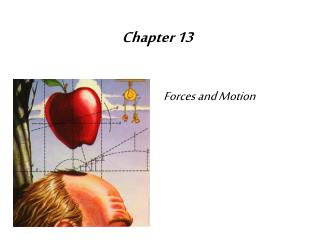DownloadDownload PresentationChapter 13

# Chapter 13

Download Presentation## Chapter 13

- - - - - - - - - - - - - - - - - - - - - - - - - - - E N D - - - - - - - - - - - - - - - - - - - - - - - - - - -
##### Presentation Transcript

1. Chapter 13 Forces and Motion

2. Gravity • Force of attraction • Dependent upon the masses of each object

3. Mass vs Weight • Mass • Amount of matter in an object • Does not change in the universe • Measured in N -- newtons • Weight • Measure of gravitational force • Varies • Measured in kg or pounds

4. Law of Universal Gravitation • All objects in the universe attract each other through gravitational force • Depends upon • Mass • Distance

5. Acceleration and Mass

6. Orbital Motion • Horizontal movement • Vertical movement

7. Falling Objects: No Air Resistance

8. Falling Objects: Air Resistance

9. Newton’s First Law • An object at rest remains at rest, and an object in motion remains in motion at constant speed and in a straight line unless acted on by an unbalanced force.

10. Inertia

11. Newton’s Second Law • The acceleration of an object depends on the mass of the object and the amount of force applied.

12. Newton’s Third Law • Whenever one object exerts a force on a second object, the second object exerts an equal and opposite force on the first.

13. Additional Terms to Know • Inertia • Terminal velocity • Free fall • Vacuum • Centripetal force